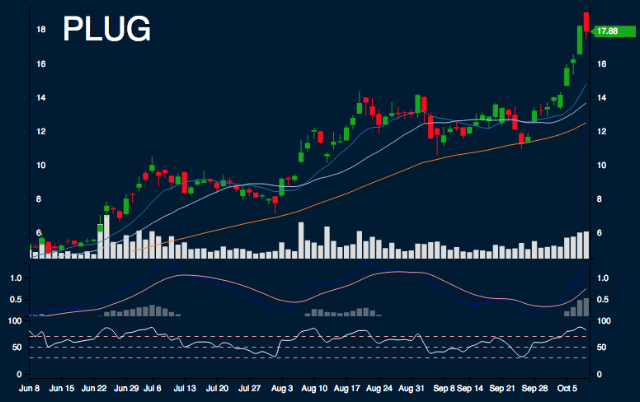#PLUG Report

Sample Size: 2 (Small Sample Size, not statistically significant); Percent Error: 5%

## Current Trend Performance

PLUG is -1.93% from monthly high, 56.88% from monthly low, 21.11% from 10 day simple moving average, 30.96% from 20 day simple moving average, and 43.23% from 50 day simple moving average.

## Forecast Ranges

Day 1:
The highs are 17.88, the lows are 16.73, and the median is 17.3. 100.0% to decrease between -13.3% - 0%.
Day 2:
The highs are 17.88, the lows are 16.15, and the median is 17.02. 100.0% to decrease between -13.3% - 0%.
Day 3:
The highs are 17.88, the lows are 14.33, and the median is 16.11. 50.0% to decrease between -26.6% - -13.3%. 50.0% to decrease between -13.3% - 0%.
Day 4:
The highs are 17.88, the lows are 15.23, and the median is 16.55. 50.0% to decrease between -26.6% - -13.3%. 50.0% to decrease between -13.3% - 0%.
Day 5:
The highs are 17.88, the lows are 13.53, and the median is 15.7. 50.0% to decrease between -26.6% - -13.3%. 50.0% to decrease between -13.3% - 0%.
Day 6:
The highs are 17.88, the lows are 10.74, and the median is 14.31. 50.0% to decrease between -39.9% - -26.6%. 50.0% to decrease between -13.3% - 0%.
Day 7:
The highs are 17.88, the lows are 11.32, and the median is 14.6. 50.0% to decrease between -39.9% - -26.6%. 50.0% to decrease between -13.3% - 0%.

## Summary

SMA: Simple Moving Average

In next 5 days, there is possible support range between 15.5 and 15.5, because of 10 day SMA. There is possible resistance range between 17.88, because of monthly high, and 20.26. Areas of uncertainty are beyond 14.72 and beyond 22.95. Minimum movement range is 13.3%.

Research conducted with Nforecast.

This is not a recommendation to buy, sell, or hold. This research is for educational, informational, and entertainment purposes. You should not construe any data or information presented by members on this blog or Nforecast LLC as investment, tax, legal, financial or other advice.